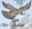Trending ▼   ResFinder# ICSE Class X Prelims 2019 : Computer Applications (The Hyderabad Public School (HPS), Begumpet, Hyderabad)

4 pages, 41 questions, 33 questions with responses, 43 total responses,30Radhika Jawaji The Hyderabad Public School (HPS), Begumpet, Hyderabad
+Fave Message
 Home > radumasup >   F Also featured on: School Page icseFormatting page ...

Pre-Board-II 2018-2019 COMPUTER APPLICATIONS Class: 10 Time: 2 hr. Date: 29 / 01 / 2019 Max Marks: 100 Answers to this paper must be written on the paper provided separately. You will not be allowed to write during the first 15 minutes. This time is to be spent in reading the question paper. The time given at the head of this paper is the time allowed for writing the answers. This paper is divided into two Sections. Attempt all questions from SECTION A and any four questions from SECTION- B. SECTION - A (40 Marks) Attempt ALL questions from the following. Question 1 a) Write a java statement to create an object of BufferedReader and accept a char input using it. b) Differentiate between double and Double. c) Which of these lines will not compile correctly? Give reason(s). short s = 20; float f=1.4; byte b = 128; char c=32; double d=1.4; byte e =0; d) Give the output of the following program segment: (Show Working) double x = 2.9, y = 2.5; System.out.println(Math.min(Math.floor(x), y)); System.out.println(Math.max(Math.ceil(x), y)); e) Identify and name the literals listed below: (i) 0.5 (ii) A (iii) false (iv) A f) How many bits/bytes are occupied by the following primitive data types? (i) long (ii) char (iii) boolean (iv) short Question 2       i) What happens when you add a double value to a String? What will be the output when the following statements get executed?  String k = "HPS"+16.25; System.out.println(k); ii) What is the length of the string returned by the call "Hyderabad".substring(3, "Hyderabad".length())?  iii) What are input and output streams?  iv) Write an equivalent Java expression for the following Mathematical expression.  3 (a+b) |a b| v) Write the conditional statement for the following decision making situation:  Provided the value of x is greater than 9, increase the value of y by 15, otherwise decrease the value of y by 36. vi) What will be the value of K when the following expression is evaluated? Determine the hierarchy of operations and show evaluation steps. Page 1 of 4Formatting page ...

Top Contributors
to this ResPaperAquaman- King o...(38)Purvam Prajapati(9)Aryan Gupta(7)Annhaliator(4)Formatting page ...Formatting page ...